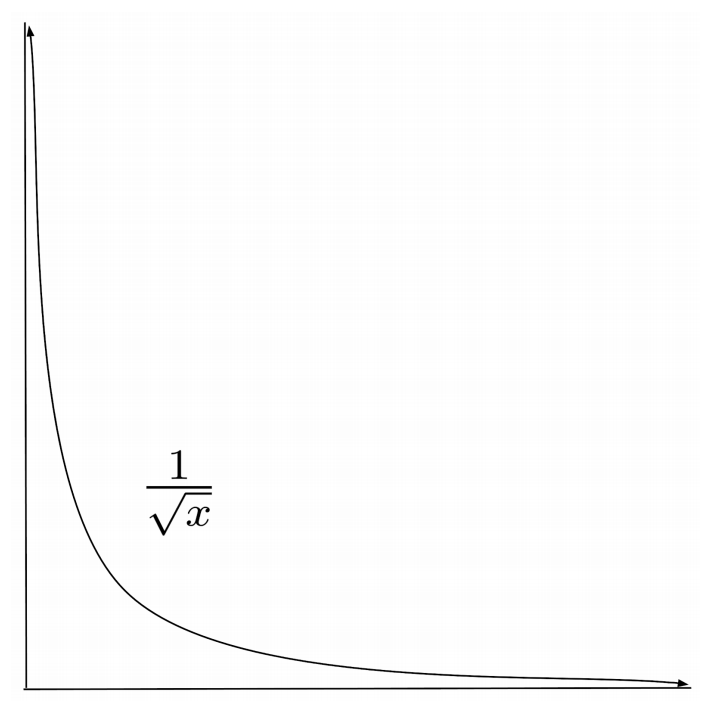# Improper Integrals

Improper integrals have bounds or function values that extend to positive or negative infinity.

This post is part of a series.

Improper integrals have bounds or function values that extend to positive or negative infinity.

For example, $\int_1^\infty \frac{1}{x^2} \, dx$ is an improper integral because its upper bound is at infinity. Likewise, $\int_0^1 \frac{1}{\sqrt{x}} \, dx$ is an improper integral because $\frac{1}{\sqrt{x}}$ approaches infinity as approaches the lower bound of integration, $0$.## Convergence

It seems intuitive that improper integrals should always come out to infinity, since an infinitely long or infinitely high function would seemingly have infinite area.

However, although this can sometimes happen, it is not always the case. In fact, both of the two improper integrals given as examples in the previous paragraph evaluate to normal, non-infinite results. As such, we say that these integrals converge.

\begin{align*} \int_1^\infty \frac{1}{x^2} \, dx &= \left[ -\frac{1}{x}\right]_1^\infty \\ &= -\frac{1}{\infty} - \left(-\frac{1}{1} \right) \\ &= 0 + 1 \\ &= 1 \end{align*}

\begin{align*} \int_0^1 \frac{1}{\sqrt{x}} \, dx &= \left[ 2\sqrt{x} \right]_0^1 \\ &= 2\sqrt{1}-2\sqrt{0} \\ &=2-0 \\ &= 2 \end{align*}

If the function decreases quickly enough as it extends out to infinity, then the area underneath it can come out to a finite number. Likewise, if a function blows up to infinity slowly enough as it approaches an asymptote, then the area underneath it can come out to a finite number.

## Divergence

Below, we integrate the function $f(x)=\frac{1}{x}$, which decreases more slowly as it extends out to infinity and blows up to infinity more quickly as it approaches its vertical asymptote $x=0$. The integrals of this function do indeed integrate to infinity. As such, we say that these integrals diverge.

\begin{align*} \int_1^\infty \frac{1}{x} \, dx &= \left[ \ln x \right]_1^\infty \\ &= \ln \infty - \ln 1 \\ &= \infty - 0 \\ &= \infty\end{align*}

\begin{align*} \int_0^1 \frac{1}{x} \, dx &= \left[ \ln x \right]_0^1 \\ &= \ln 1 - \ln 0 \\ &= 0 - (-\infty) \\ &= \infty \end{align*}

## Discontinuities within the Interval of Integration

Sometimes, a function may blow up to infinity somewhere within the interval of integration, rather than at the bounds of integration. In such a case, we have to separate the integral across its discontinuities.

For example, to compute the integral $\int_{-1}^2 \frac{1}{x^2} \, dx$, we may be tempted to ignore the singularity at $x=0$ and simply evaluate the antiderivative at the bounds. This leads us to an invalid result.

\begin{align*} \int_{-1}^2 \frac{1}{x^2} \, dx &= \left[ -\frac{1}{x} \right]_{-1}^2 \hspace{1.55cm} \mbox{(invalid)} \\ &= -\frac{1}{2} - \left( -\frac{1}{-1} \right) \hspace{.5cm} \mbox{(invalid)} \\ &= -\frac{1}{2} - 1 \hspace{1.8cm} \mbox{(invalid)} \\ &= -\frac{3}{2} \hspace{2.5cm} \mbox{(invalid)} \end{align*}

This result of negative area doesn’t make any sense, because the function $\frac{1}{x^2}$ is always positive!

In order to properly evaluate the integral $\int_{-1}^2 \frac{1}{x^2} \, dx$, we have to split it up across the singularity, into two separate integrals.

The first integral spans from $x=-1$ to $x=0$ and consequently approaches $0$ from the negative side, so its computations involve $0^-$.

The second integral spans from $x=0$ to $x=2$ and consequently approaches $0$ from the positive side, so its computations involve $0^+$.

\begin{align*} \int_{-1}^2 \frac{1}{x^2} \, dx &= \int_{-1}^0 \frac{1}{x^2} \, dx + \int_0^2 \frac{1}{x^2} \, dx \\ &= \left[ -\frac{1}{x} \right]_{-1}^{0^-} + \left[ -\frac{1}{x} \right]_{0^+}^2 \\ &= \left[ -\frac{1}{0^-} - \left( - \frac{1}{-1} \right) \right] + \left[ -\frac{1}{2} - \left( -\frac{1}{0^+} \right) \right] \\ &= \left[ -(-\infty) - 1 \right] + \left[ -\frac{1}{2} - (-\infty) \right] \\ &= ( \infty - 1 ) + \left( -\frac{1}{2} + \infty \right) \\ &= \infty + \infty \\ &= \infty \end{align*}

Now, we see that the integral actually diverges to infinity. This makes much more sense, since we know that it represents a region that contains a portion of infinite area.

Lastly, below is an example of a more complicated integral that converges.

\begin{align*} \int_0^\infty \frac{e^{-\sqrt{x}} }{\sqrt{x} } \, dx &= \left[ -2e^{-\sqrt{x}} \right]_0^\infty \\ &= -2e^{-\infty} - \left( -2e^{0} \right) \\ &= 0 - \left( -2 \right) \\ &= 2 \end{align*}

## Exercises

Evaluate the improper integrals below. (You can view the solution by clicking on the problem.)

\begin{align*} 1) \hspace{.5cm} \int_1^\infty \frac{1}{x^5} \, dx \end{align*}
Solution:
\begin{align*} \frac{1}{4} \end{align*}

\begin{align*} 2) \hspace{.5cm} \int_3^\infty \frac{1}{\sqrt{x} } \, dx \end{align*}
Solution:
\begin{align*} \infty \end{align*}

\begin{align*} 3) \hspace{.5cm} \int_5^\infty \frac{1}{x^2} \, dx \end{align*}
Solution:
\begin{align*} \frac{1}{5} \end{align*}

\begin{align*} 4) \hspace{.5cm} \int_{-\infty}^{-2} \frac{1}{(x-1)^4} \, dx \end{align*}
Solution:
\begin{align*} \frac{1}{81} \end{align*}

\begin{align*} 5) \hspace{.5cm} \int_0^1 \frac{1}{x-1} \, dx \end{align*}
Solution:
\begin{align*} -\infty \end{align*}

\begin{align*} 6) \hspace{.5cm} \int_{-3}^3 \frac{1}{(x+2)^5} \, dx \end{align*}
Solution:
\begin{align*} \infty - \infty \text{ (indeterminate)} \end{align*}

\begin{align*} 7) \hspace{.5cm} \int_\frac{3}{2}^{10} \frac{1}{\sqrt{4x-6} } \, dx \end{align*}
Solution:
\begin{align*} \sqrt{ \frac{17}{2} } \end{align*}

\begin{align*} 8) \hspace{.5cm} \int_3^\infty \frac{1}{(2x+1)^{3/2} } \, dx \end{align*}
Solution:
\begin{align*} \frac{1}{\sqrt{7}} \end{align*}

\begin{align*} 9) \hspace{.5cm} \int_0^\infty \frac{x}{(x^2+1)^2} \, dx \end{align*}
Solution:
\begin{align*} \frac{1}{2} \end{align*}

\begin{align*} 10) \hspace{.5cm} \int_0^\infty \frac{1}{\left( \sqrt{x}+1 \right)^2 \sqrt{x} } \, dx \end{align*}
Solution:
\begin{align*} 2 \end{align*}

\begin{align*} 11) \hspace{.5cm} \int_0^\infty e^{-x} \, dx \end{align*}
Solution:
\begin{align*} 1 \end{align*}

\begin{align*} 12) \hspace{.5cm} \int_0^\infty \frac{1}{1+x^2} \, dx \end{align*}
Solution:
\begin{align*} \frac{\pi}{2} \end{align*}

This post is part of a series.

Tags: